Product of successive primes in Cunningham chain of the second kind satisfying congruence conditions is Poulet number

Statement

Supposeis an odd prime number such thatis also a prime number. Then, the number:is a Poulet number (i.e., it is a Fermat pseudoprime to base 2) if and only if.

Note that the condition thatare both primes means that they are consecutive members of a Cunningham chain of the second kind.

Particular cases

We give here the smallest few values of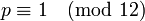such thatare both primes, and the corresponding Poulet numbers.Corresponding Poulet number (Fermat pseudoprime to base 2)
37 73 2701
97 193 18721
157 313 49141
229 457 104653

Facts used

1. Fermat's little theorem
2. Euler's criterion (for quadratic residue)
3. Congruence condition for two to be a quadratic residue

Proof

Given:is a prime number such thatis also a prime number. Let.

To prove:if and only if.

Proof: We have: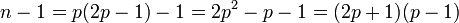Thus: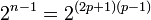We thus have:

Step no. Assertion/construction Facts used Given data used Previous steps used Explanation
1is congruent to 1 mod, or equivalently,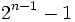is divisible by. Fact (1)is prime The order of 2 mod p divides, which divides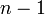. More explicitly: [SHOW MORE]
2is congruent to 1 or -1 mod, depending on whether 2 is a quadratic residue mod(1 if it is, -1 if it isn't) Facts (1), (2)is prime [SHOW MORE]
3is congruent to 1 or -1 mod, with 1 iffis congruent to 1 mod 12. Fact (3)are primes,is odd Step (2) [SHOW MORE]
4is congruent to 1 modiff.Steps (1), (3) Step-combination direct.